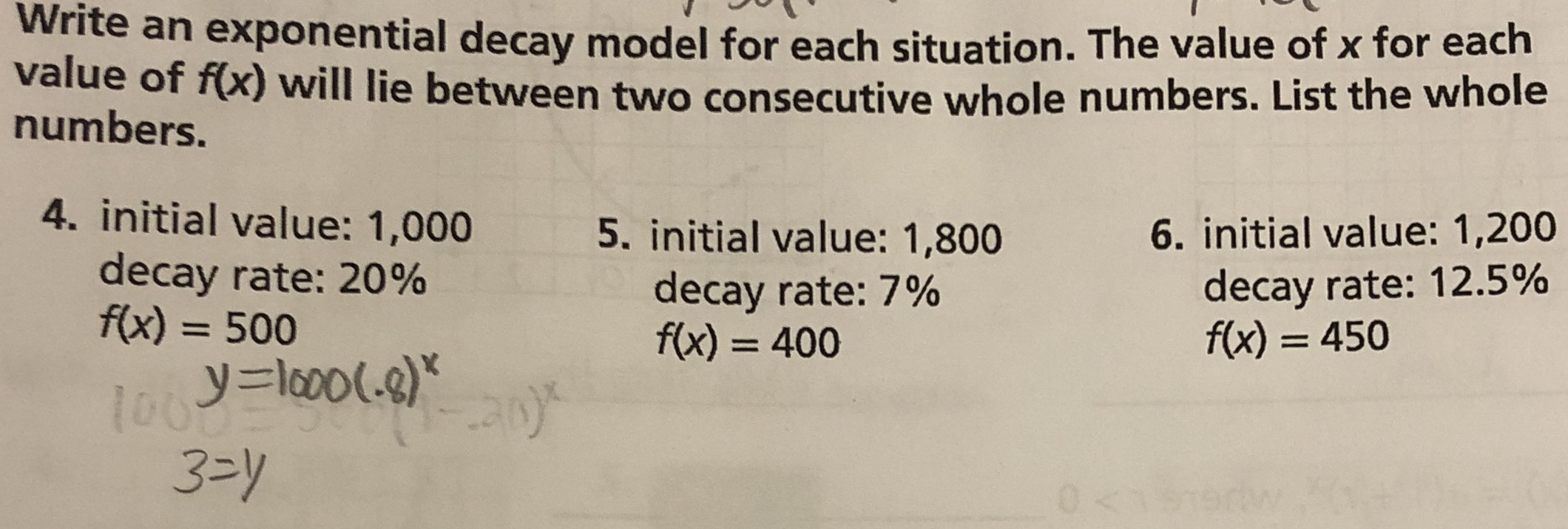### ¿Todavía tienes preguntas de matemáticas?

Pregunte a nuestros tutores expertos
Algebra
PreguntaWrite an exponential decay model for each situation. The value of $$x$$ for each value of $$f ( x )$$ will lie between two consecutive whole numbers. List the whole numbers.

4. initial value: $$1,000$$ decay rate: $$20 \%$$

$$f ( x ) = 500$$

5. initial value: $$1,800$$ decay rate: $$7 \%$$

$$f ( x ) = 400$$

6. initial value: $$1,200$$ decay rate: $$12.5 \%$$

$$f ( x ) = 450$$

$$3,4\\20,21\\7,8$$